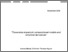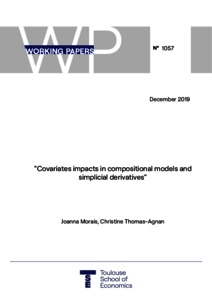# Covariates impacts in compositional models and simplicial derivatives

Thomas-Agnan, Christine and Morais, Joanna (2019) Covariates impacts in compositional models and simplicial derivatives. TSE Working Paper, n. 19-1057, ToulousePreview
Text

## Abstract

In the framework of Compositional Data Analysis, vectors carrying relative information, also called compositional vectors, can appear in regression models either as dependent or as explanatory variables. In some situations, they can be on both sides of the regression equation. Measuring the marginal impacts of covariates in these types of models is not straightforward since a change in one component of a closed composition automatically affects the rest of the composition. J. Morais, C. Thomas-Agnan and M. Simioni [Austrian Journal of Statistics, 47(5), 1-25, 2018] have shown how to measure, compute and interpret these marginal impacts in the case of linear regression models with compositions on both sides of the equation. The resulting natural interpretation is in terms of an elasticity, a quantity commonly used in econometrics and marketing applications. They also demonstrate the link between these elasticities and simplicial derivatives. The aim of this contribution is to extend these results to other situations, namely when the compositional vector is on a single side of the regression equation. In these cases, the marginal impact is related to a semi-elasticity and also linked to some simplicial derivative. Moreover we consider the possibility that a total variable is used as an explanatory variable, with several possible interpretations of this total and we derive the elasticity formulas in that case.

Item Type: Monograph (Working Paper) English December 2019 Toulouse compositional regression model, marginal effects, simplicial derivative, elasticity, semi-elasticity. C10 - GeneralC25 - Discrete Regression and Qualitative Choice Models; Discrete RegressorsC35 - Discrete Regression and Qualitative Choice Models; Discrete RegressorsC46 - Specific Distributions; Specific StatisticsD12 - Consumer Economics - Empirical AnalysisM31 - Marketing B- ECONOMIE ET FINANCE TSE-R (Toulouse) Université Toulouse 1 Capitole UT1 06 Dec 2019 13:31 06 Dec 2019 13:31 oai:tse-fr.eu:123765 http://publications.ut-capitole.fr/id/eprint/33560View Item